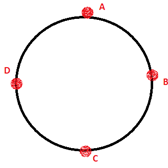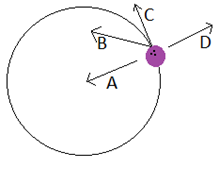Physics
Easy

Question

# A boy moves a tied stone above his head in circles, time period of circular motion is 2 sec and it forms a circle of 20 cm radius. What is the speed of rotation?

## 0.5 m/s0.6 m/s0.7 m/s0.8 m/sHint:

## The correct answer is: 0.7 m/s

Physics

Physics

Physics

### What is the direction of the velocity at point mentioned if the ball is revolving clockwise.At point BPhysics

Physics

Physics

### Which of the following depicts the direction of velocity of the ball circulated around a point?Physics

Physics

Physics

### What is the relation between radius, velocity, and time period?Physics

Physics

Physics

### If there is a change in the rate of rotation, then the circular motion will be _______.Physics

Physics

Physics

### What is the centripetal force for an object of mass 50 Kg with the following specifications moving in a uniform circular motion.Distance between the object and the axis point is 100 cm. Object’s speed is 40 m/s.#### With Turito Foundation.#### Get an Expert Advice From Turito.